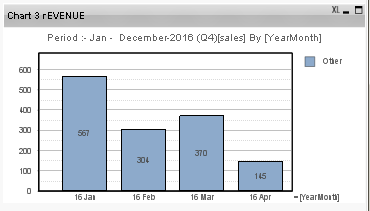# QlikView Scripting

Discussion Board for collaboration on QlikView Scripting.

Announcements

#### Breathe easy -- you now have more time to plan your next steps with Qlik! QlikView 11.2 Extended Support is now valid through December 31, 2020. Click here for more information.

Valued Contributor II

## aggr(sum(total <\$(ColumnDim_p)> sales) How to replace with rEVENUE ?

Hi All

I have expression as below work fine for chart 1:-

sum({\$<year = {"<=\$(=max(year)) >=\$(=max(year)-12)"}, month = {"<=\$(=max({<year={\$(=max(year))}>} month))"}>} \$(ColumnDim51)/1000/\$(Columndim89))

I also have expression below work fine for chart 2 :-

sum({\$<year = {"<=\$(=max(year)) >=\$(=max(year)-12)"}, month = {"<=\$(=max({<year={\$(=max(year))}>} month))"}>} \$(ColumnDim601)/1000/\$(Columndim89))

when user select Dim2 = rEVENUE , I have convert the above expression to below , and this expression i insert at Chart 3 :-

sum({\$<year = {"<=\$(=max(year)) >=\$(=max(year)-12)"}, month = {"<=\$(=max({<year={\$(=max(year))}>} month))"}>} rEVENUE={rEVENUE}>}Amount/1000/\$(Columndim89))

Chart 3 Dimension expression as below :-

=if(aggr(sum(sales),\$(ColumnDim101)) / aggr(sum(total <\$(ColumnDim_p)> sales), \$(ColumnDim101)) > 0.00001, \$(ColumnDim101), ' Other')

Can some one advise me how to change the above expression sales to rEVENUE ?

So that chart 3 is able to display the bar.

Paul

1 Solution

Accepted Solutions
Contributor III

## Re: aggr(sum(total <\$(ColumnDim_p)> sales) How to replace with rEVENUE ?

Hi Paul,

I was checking your expression, please check the characters in bold and red:

sum({\$<year = {"<=\$(=max(year)) >=\$(=max(year)-12)"}, month = {"<=\$(=max({<year={\$(=max(year))}>} month))"}>} rEVENUE={rEVENUE}>}Amount/1000/\$(Columndim89))

I just remove those characters (I also added single quotes to your rEVENUE value):

sum({\$<year = {"<=\$(=max(year)) >=\$(=max(year)-12)"}, month = {"<=\$(=max({<year={\$(=max(year))}>} month))"}, rEVENUE={'rEVENUE'}>}Amount/1000/\$(Columndim89))

The chart displays the following information, but I'm not sure if this fills your needs:Regards,

-- Karla

3 Replies
Valued Contributor II

My QV Doc

Contributor III

## Re: aggr(sum(total <\$(ColumnDim_p)> sales) How to replace with rEVENUE ?

Hi Paul,

I was checking your expression, please check the characters in bold and red:

sum({\$<year = {"<=\$(=max(year)) >=\$(=max(year)-12)"}, month = {"<=\$(=max({<year={\$(=max(year))}>} month))"}>} rEVENUE={rEVENUE}>}Amount/1000/\$(Columndim89))

I just remove those characters (I also added single quotes to your rEVENUE value):

sum({\$<year = {"<=\$(=max(year)) >=\$(=max(year)-12)"}, month = {"<=\$(=max({<year={\$(=max(year))}>} month))"}, rEVENUE={'rEVENUE'}>}Amount/1000/\$(Columndim89))

The chart displays the following information, but I'm not sure if this fills your needs:Regards,

-- Karla

Valued Contributor II

## Re: aggr(sum(total <\$(ColumnDim_p)> sales) How to replace with rEVENUE ?

Hi Karla

Thank you for your advise . I have test it does give the chart. But due to my dimension expression have some issue , i need to solve it .

Paul

Community Browser